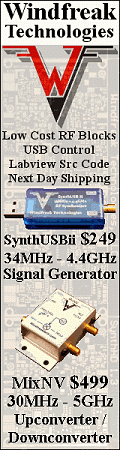Please Support RF Cafe by purchasing my  ridiculously low−priced products, all of which I created.

RF Workbench  (shareware)# Quarter-Wave (λ/4-wave) Coaxial Resonators

Quarter-wave (λ/4-wave) coaxial resonators are constructed by shorting the center conductor of a coaxial cable to the shield at the far end of the circuit. The length of the cable is exactly λ/4 at the desired resonant frequency. A short circuit is transformed to an open circuit a quarter wavelength away, so when the λ/4-wave coaxial resonator is part of an oscillator circuit, it electrically is not even present (Z~∞); however, whenever the frequency of the oscillator attempts to go above or below the resonator's center frequency (due to load changes, temperature changes, etc.), the λ/4-wave section looks like a low impedance that works to attenuate other frequency components. It acts like a parallel tuned L/C tank circuit. The advantage of a λ/4-wave coaxial resonator over a tuned L/C tank circuit is the much higher quality factor, "Q."

Coaxial resonators are often made from sections of normal coaxial cable (both flexible and semi-rigid), but many form factors are available that are manufactured for specific frequencies and mount on printed circuit boards, or have coaxial connectors installed on both ends. Amateur radio hobbyists often use λ/4-wave resonators to filter (trap) interference outside the working band of the radio operation. The nice thing about λ/4-wave trap it that is can be connected inline with the antenna feed cable using a simple "T" adapter (remember, it is effectively not even there at the center frequency).

Quality factor of a λ/4-wave coaxial resonator is determined by the ratio of the center frequency to the 3 dB power bandwidth, as shown in the figure below. A set of equations is then presented for calculating the necessary λ/4-wave coaxial resonator parameters.Quality Factor (Q) of a λ/4-Wave Coaxial ResonatorDimension Reference for λ/4-Wave Coaxial Resonator EquationsQc Qcc Qd Q a b  f μ σ εr n Z0 Zopt = conductor contribution to unloaded Q= conductor contribution to unloaded Q for copper   conductors with optimum b/a ratio of 3.59112= dielectric contribution to unloaded Q= unloaded quality factor of a λ/4-wave resonator,   including conductor and dielectric losses= outside radius of inner conductor (m)= inside radius of outer shield (m) = resonant frequency (Hz)= permeability of the conductor (H/m)= conductivity of the conductor (mho/m)= relative dielectric constant between a and b= resonator mode (# of 1/4 wavelengths at resonance)= characteristic impedance of coaxial structure (Ω)= characteristic impedance that yields the highest   theoretical value of Q (b/a = 3.59112) λ

Note: These are commonly available equations; my source was Vizmuller

Related Pages on RF Cafe